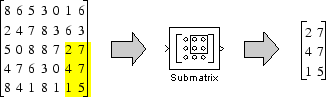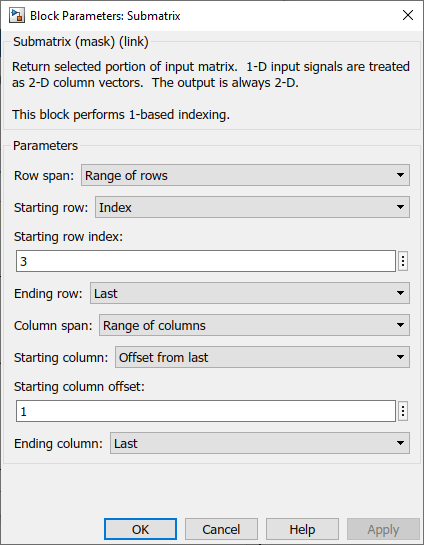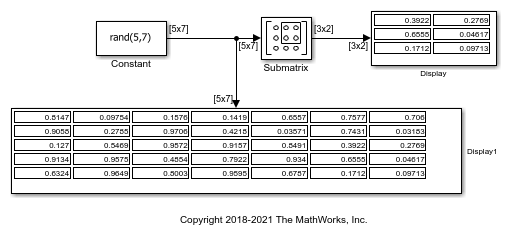# Extract 3-by-2 Submatrix from Input Signal

This example shows how to use the Submatrix block to extract a 3-by-2 submatrix from the lower-right corner of a 5-by-7 input matrix. The following figure illustrates the operation of the Submatrix block with a 5-by-7 input matrix of random integer elements, `randi([0 9],5,7)`.Here are the settings used for the `Submatrix` block in this example.There are often several possible parameter combinations that you can use to select the same submatrix from the input. For example, in the case of a 5-by-7 input matrix, instead of specifying `Last` for Ending column, you could select the same submatrix by specifying:

• Ending column = `Index`

• Ending column index = `7`

Open and simulate the model. You can see that the 3-by-2 submatrix from the lower-right corner of a 5-by-7 input matrix has been extracted.